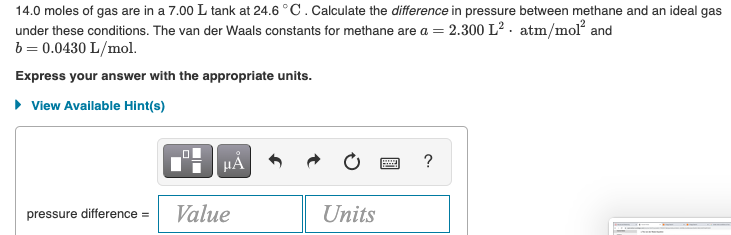Home / Expert Answers / Chemistry / 14-0-moles-of-gas-are-in-a-7-00-mathrm-l-tank-at-24-6-circ-mathrm-c-pa637

# (Solved): $$14.0$$ moles of gas are in a $$7.00 \mathrm{~L}$$ tank at $$24.6{ }^{\circ} \mathrm{C}$$. ...$$14.0$$ moles of gas are in a $$7.00 \mathrm{~L}$$ tank at $$24.6{ }^{\circ} \mathrm{C}$$. Calculate the difference in pressure between methane and an ideal gas under these conditions. The van der Waals constants for methane are $$a=2.300 \mathrm{~L}^{2} \cdot \mathrm{atm} / \mathrm{mol}^{2}$$ and $$b=0.0430 \mathrm{~L} / \mathrm{mol}$$ Express your answer with the appropriate units.

We have an Answer from Expert